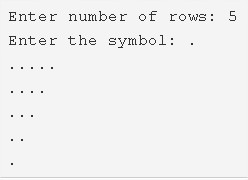# C Program Inverted Right Triangle Star Pattern – Pattern Programs

C program to print an inverted right triangle star pattern – In this article, we will detail in on the multitude of methods to print an inverted right triangle star pattern in C programming.

Suitable examples and sample programs have also been added so that you can understand the whole thing very clearly. The compiler has also been added with which you can execute it yourself.

The methods used in this piece are as follows:

• Using For Loop
• Using While Loop
• Using Do-While Loop

A Right triangle is a very common type of two-dimensional figure used in the field of geometry. It is a type of a triangle which has one angle equal to 90 degrees.

The sum of squares of the sides adjacent to the right angle is equal to the square of the side opposite to the right angle.Firstly, as per the given example, you need to clear the number of rows of the pattern to be printed.

The number of rows stipulated for this pattern in the example is 5.

Then, you need to enumerate the symbol you need to use to print the pattern.

The symbol ‘*’ is chosen to print the Inverted Right Triangle Star Pattern.

Hence, the pattern is printed consequently as per the code.

Thus, the manifold ways to do the same in C programming are as follows:

## Using For Loop

1. Read the entered number of rows, symbols using scanf(), getchar() functions and store those values into the variables n, ch.

2) The outer for loop iterates through rows with the structure for(i=n;i>0;i–).

3) The inner for loop iterates through columns with the structure for(j=0;j<i;j++).

The loop prints the symbol which is entered by the user.i.e each row contains i symbols.

4) Cursor comes to next line after each row(go to step 2).

5)In the given example n=5.

The 1st for loop iterates through rows. The 2nd for loop prints 5 stars at 1st row,4 stars at 2nd row,3 stars at 3rd row,2 stars at 4th row, one star at 5th row.

Output:

### Using While Loop

1. The outer while loop iterates through rows until the condition i>0 becomes false.

2) The inner while loop iterates through columns until the condition j<i becomes false.

Inner loop prints symbol,j value increased by 1.

3) Cursor comes to next line. i value decreased by 1.

Output:

#### Using Do-While Loop

1. The outer do-while loop iterates through rows.

2) The inner do-while loop iterates through columns.

Prints symbol.

j value increased by 1.

Checks the condition j<i. If the condition is true then loop iterates again. Repeats until the condition fails.

3) Cursor comes to next line.i value decreased by1.

4) Checks the condition i>0 of the outer do-while loop. If the condition is true then loop iterates again. Repeats until the condition fails.

Output:

x

## C Program To Print Number Of Days In A Month | Java Tutoring

C program to input the month number and print the number of days in that ...